# Making a rhythm game with bleeding-edge webWhile continuing to experiment with Web Audio API and GLSL, I've made a game called Timelapse for js13kgames (an HTML5 game competition where entries must be less than 13 kb zipped).

This article is a postmortem overview of my game development which will try to explain what was my game mecanism ideas and show you some interesting parts with screenshots, audios and source code snippets.

## The Game

The game intends to work on Desktop and Mobile. However, Chrome is recommended (Firefox Aurora also supports it but audio is a bit wrong, but Mozilla devs should improve this soon). Today, it works on Android Chrome Beta on a Nexus 4, unfortunately with some clicks in the audio (Web Audio API is bleeding-edge).

## Experimenting with stuff

Last months, I've been playing with Web Audio API and released a few experiments like Beez, FM Synthesis and Zound.

My game development started last weekend as an experiment, I tried to make some dubstep-like sound, starting with a "Wob Wob Wob" sound.

I also started to dig into glsl.heroku.com, I definitely wanted to make some cool and unusual graphics with glitchy style to fit with dubstep audio part, I unfortunately hadn't enough deepen this glitchy part as I would have liked.

GLSL quite fits this need: it mays look strange and hard code language as a start but it's very easy and free to do anything with it. I used my glsl.js wrapper to easily have a shader rendering the whole Canvas.

GLSL is a totally different way of thinking the rendering: the main principle is to define a function which returns a Color for a given Position. I use to call it "Functional Rendering" in opposite to "Procedural Rendering". I'll talk again about those concepts soon.

I started my graphics by forking this very interesting glow effect.

## Prototyping the game ideas

Then I started to really think about the game I could do, I sketched some game mecanisms and thought about the gameplay.

My game was designed to be a one-button DDR-like rhythm game with the main idea that the user controls the speed (the BPM, beats per minute) of the song. I wanted an inertia system: tap a bit early and your song will speed up, tap a bit late and the song will slow down. This speed freedom isn't without constraints: You can reach a gameover if your speed isn't enough fast, or contrariwise if it is too fast (like a overheat).

The game is basically about SPACE-typing on each beat, but also introduce some freestyle "dubstep" phase (It's not really dubstep though!): the gameplay is either typing on the key like hell or holding and releasing some "riff".

The score mecanisms give good scores for very precise beats and will be negative for very bad/loss rhythms. During the freestyle section, each action gives a score, also each riff (holding the button for more than 1 tick) gives a score. Making small riffs has been designed to give more points that a very long riff so you have to find the good balance. (The score also increases at the end of each freestyle phase).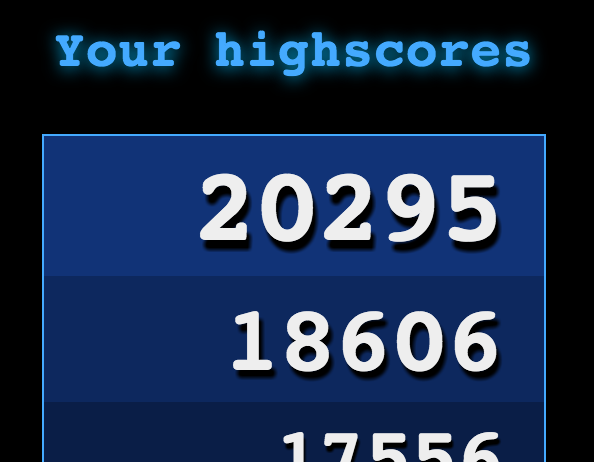I also had to find a game end, I first thought about trying to make the game harder and harder but it wasn't trivial to make because I wanted to keep my "player is free to take any speed he wants" idea.

Instead, I chose to limit the game time by one minute, which makes my game a psychedelic rush game if you want a good score: A good strategy to make a good score is to first speed up the song inertia as fast as possible, and then keep the rhythm on an high BPM.

That mecanism is interesting because it is also harder to make precise scores on higher speed, it can even be risky (reaching the BPM limit, failing some beats), the player has to find the speed it fits the most!

## The game experience

I wanted my game experience to be both on the graphics and on the audio aspects: you have both a feedback on your actions with the graphics using a color ( green=good, yellow=meh, red=bad ) and with the audio (different sound depending on the rate of the action).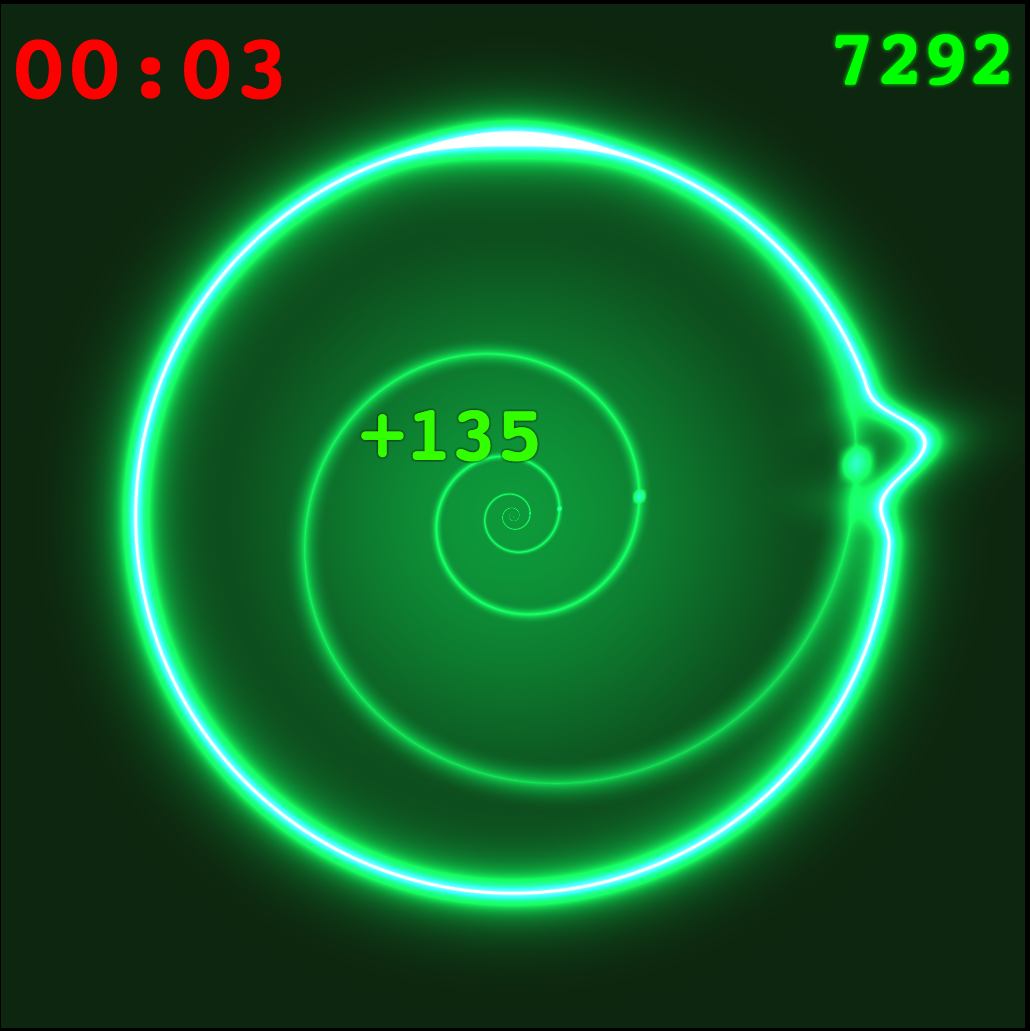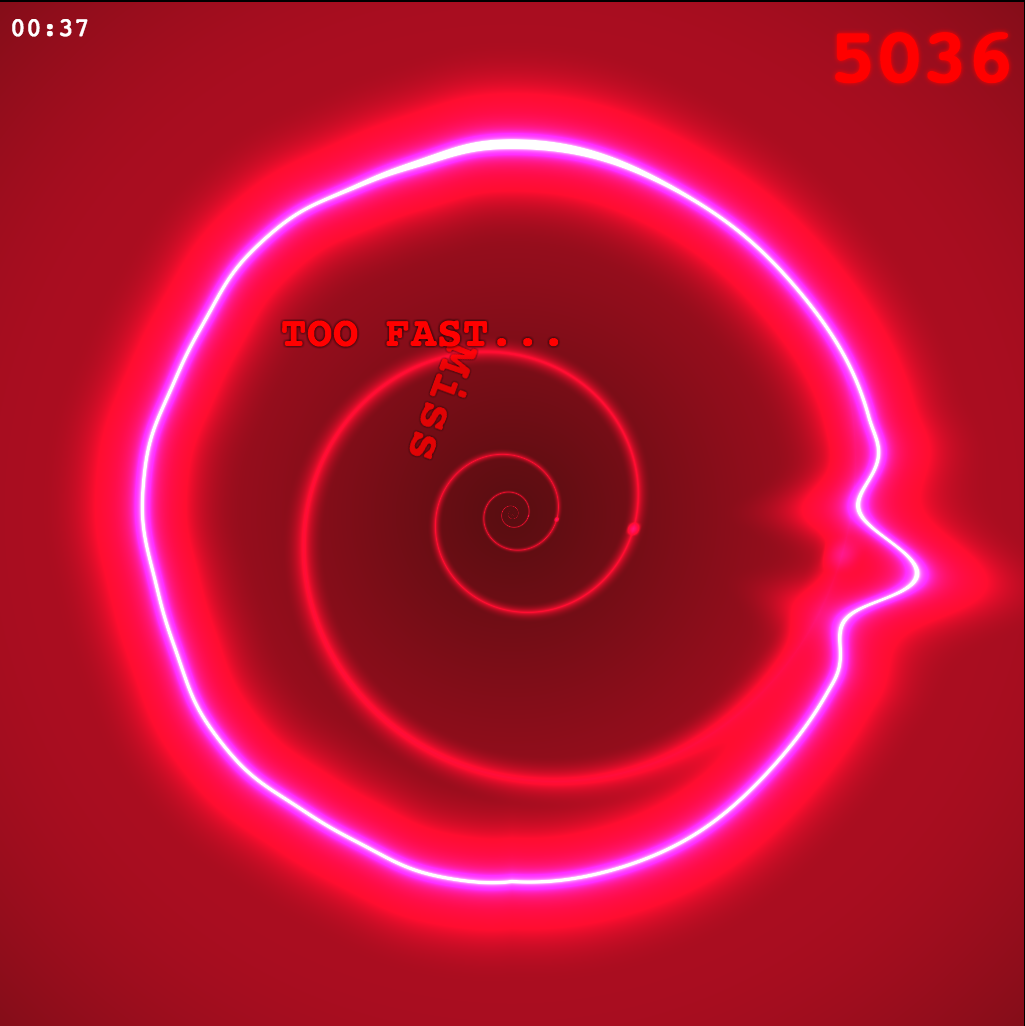The audio BPM is also graphically visualized using a circle with a rotating pulse which also helps you on the rhythm.

During the freestyle phase, the circle turns fully highlighted and the audio "wob wob wob" part is playing. Each user freestyle "riff" (hold a note) will randomly change the delay of a "repeater", an important part on the audio of that section I will discuss in the Audio Section.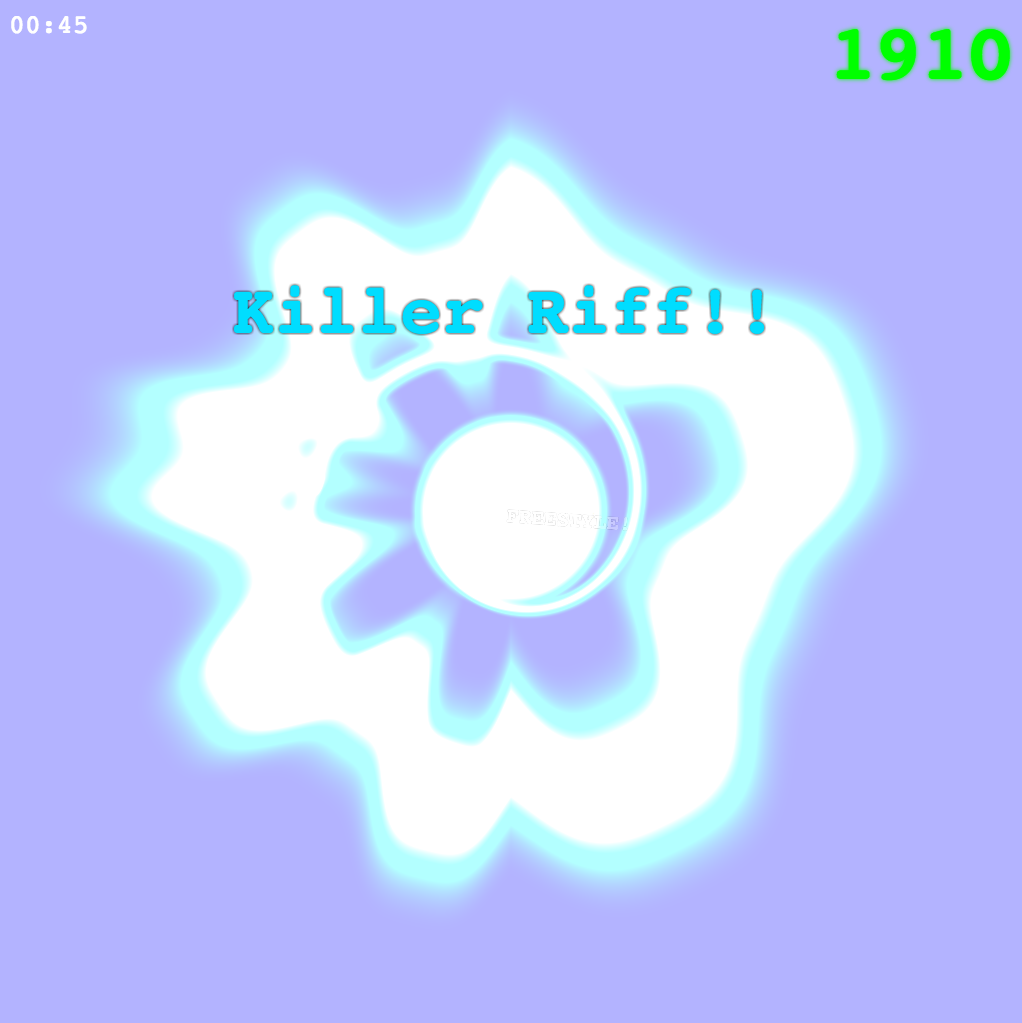If the player runs the song too fast, an overheat happens and the circle turns very light:On the contrary, it turns very dark and glitchy when the BPM is very slow: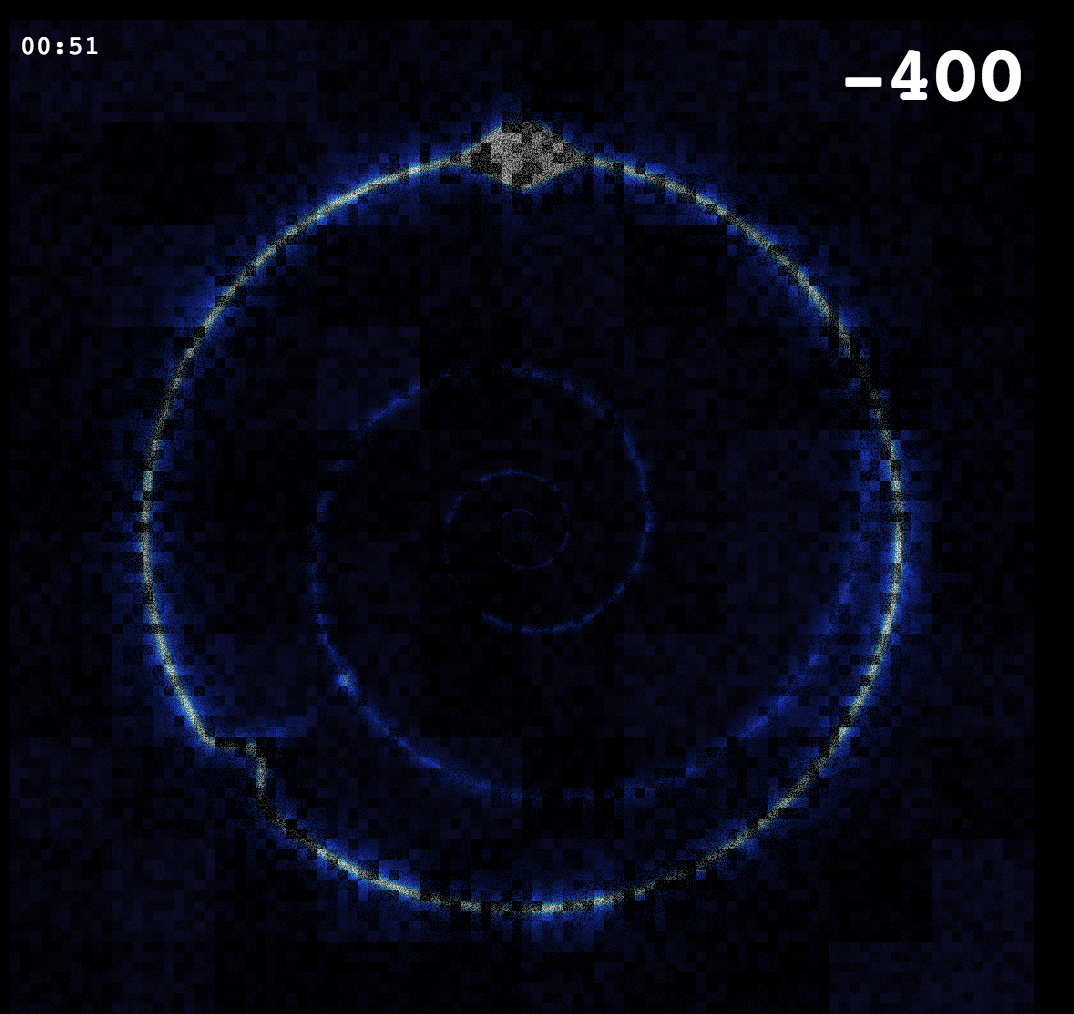As described in the specification, the Web Audio API is an audio routing graph composed of low level audio nodes. Using it raw can be quite verbose, I've made my own reusable component using those nodes. My convention is to use Javascript constructor for those components and to have a "inp" and an "out" AudioNode field.

First I create a `ctx` AudioContext (works for Chrome & Firefox Aurora):

``````var ctx = new (window.AudioContext || window.webkitAudioContext)();
``````

This `ctx` variable now offers all methods to work with sound.

### Global effects: Reverbation, Compressor

Web Audio API have a Convolver node which allows to make diverse audio effects like reverbation, which is basically emulating your song played in a room. You can find more information here.

I've used a simple Reverb effect to pass the whole sound. This simple reverb implementation can be found on https://github.com/web-audio-components/simple-reverb.

Another very important brick of the Audio graph is the Compressor. The Web Audio API have a built-in Compressor with some parameters.

A compressor dynamically adapts the input sound to a normalized output. It ensures the output is not distorted (saturated because amplitude is too high) or inaudible because too low. In other words, it consists of dynamically raise the volume if the input is lower, and decrease the volume if the input is higher, that a given rate.

Here is the global audio setup I've used as an output for all different sounds of the song:

``````  var out = ctx.createGain(); // My global output
var outCompressor = ctx.createDynamicsCompressor();
var reverb = new Reverb(0.5);
out.gain.value = 0; // We will increase the main volume when the song starts
out.connect(reverb.inp);
reverb.out.connect(outCompressor);
outCompressor.connect(ctx.destination);
``````

### Ambiant sounds

I've used a soft sine Oscillator and some Noise generator (protected by a bandpass Filter) for the ambiant sound. That gives more depth to the song.

It was also used to give more audio feedback on the gameplay:

• The Oscillator frequency follows the BPM (goes higher in frequency with the song speed).
• The BPM also affects the frequency of a LFO which oscillate the volume of the Noise to make an helicopter-like sound.
• The Oscillator is fastly detuned on each user action, and especially if the user tap too early it will produce a "bip" like you can hear in the following Soundcloud.
• Finally, a second noise passed into a highpass filter will be louder if the player is in danger (BPM is too slow or too fast). (we won't show the code for this one)

I have muted all other sounds to make you hear only the ambiant sound when speeding up the song up to a gameover:

The Noise component:

``````  function Noise () {
// Here we loop on a 2s noise buffer, it is more efficient that generating on the fly
var bufferSize = 2 * ctx.sampleRate,
noiseBuffer = ctx.createBuffer(1, bufferSize, ctx.sampleRate),
output = noiseBuffer.getChannelData(0);
for (var i = 0; i < bufferSize; i++) {
output[i] = Math.random() * 2 - 1;
}
var whiteNoise = ctx.createBufferSource();
whiteNoise.buffer = noiseBuffer;
whiteNoise.loop = true;

var gain = createGain();
whiteNoise.connect(gain);

gain.connect(filter);
filter.type = "lowpass"; // Generally lowpass, but can be overrided

this.white = whiteNoise;
this.gain = gain;
this.out = this.filter = filter;
}

Noise.prototype = {
start: function (time, duration) {
this.white.start(time, 0, duration);
}
};
``````

Here is some code I used for making the ambiant sound:

``````  var bpmOsc2mult = 3;
var bpmNoiseMult = 10;
var noiseBpmGain = ctx.createGain();
noiseBpmGain.connect(out);
var noiseBpm = new Noise();
noiseBpm.out.connect(noiseBpmGain);
noiseBpm.start(0);
noiseBpm.gain.gain.value = 0.2;
noiseBpm.filter.type = "bandpass";
noiseBpm.filter.Q.value = 20;
noiseBpm.filter.frequency.value = 0;

var bpmNoiseLfoMult = 0.05;
var bpmNoiseLfoPow = 1.3;
var lfoBpm = ctx.createOscillator();
lfoBpm.start(0);
var lfoBpmGain = ctx.createGain();
lfoBpmGain.gain.value = 0.8;
lfoBpm.connect(lfoBpmGain);
lfoBpmGain.connect(noiseBpmGain.gain);

var osc2 = new OscGain();
osc2.type = "sawtooth";
osc2.osc.frequency.value = vars.bpm * bpmOsc2mult;
osc2.osc.detune.value = 5;
osc2.gain.gain.value = 0.1;
osc2.out.connect(out);
osc2.start(0);
``````

### NOTES

To easily define melodies, I first define "NOTES", a map of `note -> frequency`. For instance `NOTES.A4` is `440` Hz:

``````var NOTES = (function () {
var notes = {};
var toneSymbols = "CcDdEFfGgAaB";
function noteToFrequency (note) {
return Math.pow(2, (note-69)/12)*440; // Beauty of audio math!
};
for (var octave = 0; octave < 8; ++octave) {
for (var t = 0; t < 12; ++t) {
notes[toneSymbols[t]+octave] = noteToFrequency(octave * 12 + t);
}
}
return notes;
}());
``````

My convention here is to use a cap for major notes like `D` and no cap for minor notes like `d` (the black keys on a Piano). The following number is the octave. Notes are defined with two characters: Like A1, C2, B3, a4, ... You will see that's quite convenient to use the `with(NOTES){ ... }` syntax.

### FM Synth "bass" melody

I used some FM synth for making the main "bass" melody:

First, I made a "OscGain" and a "FM" components.

``````  function OscGain (t) {
this.osc = ctx.createOscillator();
if (t) this.osc.type = t;
this.out = this.gain = ctx.createGain();
this.osc.connect(this.gain);
}
OscGain.prototype = {
start: function (time, duration) {
this.osc.start(time, 0, duration);
}
};

function FM () {
OscGain.call(this);
this.mod = new OscGain();
this.mod.out.connect(this.osc.frequency);
}
FM.prototype = {
start: function (time, duration) {
this.osc.start(time, 0, duration);
this.mod.start(time, duration);
}
};
``````

And used this melody:

``````with (NOTES) {
bassMelo = [G4,D4,F4,C4];
}
``````
``````  // Usage for the bass:
var bass = new FM();
bass.out.connect(out);
function tick (i, time) {
// ...
// Change the note each 4 tick
var oscFreq = bassMelo[Math.floor(i/4) % bassMelo.length];
bass.osc.frequency.value = oscFreq * 2.0;
bass.mod.osc.frequency.value = oscFreq * 0.5;
bass.mod.gain.gain.value = oscFreq * 0.5;
// ...
}
``````

N.B.: The modulator frequency is 1/4 of the oscillator frequency which gives a cool bass sound.
Also, That tick function is called at a (variable) frequency of `60 / BPM` Hz (BPM means Beat Per Minute, here it's more a Tick Per Minute) with the tick number `"i"` and the tick time `"time"`.

### FM Synth "main" melody

This "main" synth is also a Frequency Modulation, but using a 3/4 ratio on the modulator frequency, and with an envelope on each notes.

``````with (NOTES) {
melo1 = [E3,G3,D3,G3,E3,A3,C3,G3];
melo2 = [E3,B3,D3,G3,E3,C4,C3,D3];
}
``````

Making an envelope consists of scheduling the amplitude through time with a Gain. See my FM Article.

``````  function envelope (gainNode, time, volume, duration, a, d, s, r) {
var gain = gainNode.gain;
gain.cancelScheduledValues(0);
gain.setValueAtTime(gain, 0, time);
gain.linearRampToValueAtTime(volume, time + a);
gain.linearRampToValueAtTime(volume * s, time + a + d);
gain.setValueAtTime(volume * s, time + a + d + duration);
gain.linearRampToValueAtTime(0, time + a + d + duration + r);
}
``````

Also, the melody periodically switch into "arpeggio note" with this function:

``````  var DELTAS = [
Math.pow(2, 0),
Math.pow(2, 1),
Math.pow(2, 2)
];

function applyArpeggio (freqParam, baseFreq, time, duration, arpDuration, deltas) {
if (!deltas) deltas = DELTAS;
var length = deltas.length;
var ranges = [];
cancelScheduledValues(freqParam, 0);
for (var t = 0, i = 0; t <= duration; t += arpDuration, i = (i+1) % length) {
setValueAtTime(freqParam, baseFreq * deltas[i], time + t);
}
}
``````

The Arpeggio effect is about fastly changing some octaves higher (like C3,C4,C5,C3,C4,C5,... very fastly). I've keeped that "deltas" a parameter to try other arpeggios, I've only used `[1,2,4]` multiplicators in the game.

Indeed, thanks to the magic of audio math, incrementing the octave means multipling the frequency by 2, more generally increment by N octaves means multiplying by `2 ^ N`.

Finally, here is "meloNote", the function which triggers one melody note.

``````  function meloNote (noteFreq, time, arpeggio, metallic) {
var fm = new FM();
var duration = 0.3;
var release = 0.1;
fm.osc.type = "triangle";
fm.osc.frequency.value = 4 * noteFreq;
fm.mod.osc.frequency.value = 3 * noteFreq;
fm.mod.osc.type = "sine";
fm.out.connect(meloOut.inp);
setTimeout(function () {
fm.out.disconnect(meloOut.inp);
}, 1000);
startNode(fm, time, 0, 1);
arpeggio && applyArpeggio(fm.osc.frequency, 4 * noteFreq, time, duration+release, 0.025);
envelope(fm.gain, time, 0.5, duration,
0.01, 0.02, 0.6, 0.2);
envelope(fm.mod.gain, time, 4 * noteFreq * metallic, duration,
0.05, 0.1, 0.6, 0.2);
}
``````

N.B. The metallic parameter is a parameter from 0 to 1 to give a more metallic sound. It changes the modulator intensity. In fact, that 3/4 ratio on the FM is the reason metallic sound.

This function is called each tick with a new note:

``````function tick (i, time) {
// ...
var r = risk(); // How the player is in danger
var metallic = 0.4 * r + 0.3 * smoothstep(-1, 1, Math.cos(Math.PI * i / 16));
var melo = i % 16 < 8 ? melo1 : melo2;
var octave = i % 32 < 16 ? 0 : 1;
var m = melo[i % 8] * (1 << octave);
meloNote(m, time, meloIsArpeggio, metallic);
// ...
}
``````

### Repeater of freestyle part

A "repeater" with random delay add crazyness in the freestyle section. The delay time is randomly changed each time you hold the key so it gives cool feedback.

The Repeater is made of a Delay piped in a Gain and piped back in the delay input to produce feedback (echo). A particularity of this component is the input Gain is also the output Gain.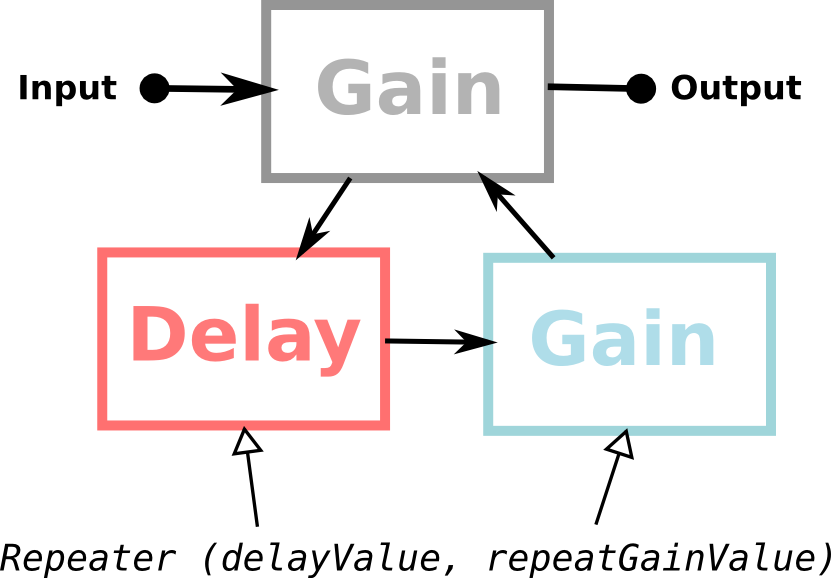Implementation of a Repeater:

``````  function Repeater (delayValue, repeatGainValue) {
var out = ctx.createGain();
var delay = ctx.createDelay(1); // The Max Delay
delay.delayTime.value = delayValue;
out.connect(delay);
var repeatGain = ctx.createGain();
repeatGain.gain.value = repeatGainValue;
delay.connect(repeatGain);
repeatGain.connect(out);
this.delay = delay;
this.repeater = repeatGain;
this.gain = this.inp = this.out = out;
}
``````

### Playing with Stereo

Doing stereo with Web Audio API can be a bit verbose without wrapping it, here is the Stereo component:

``````  function Stereo (left, right) {
var merger = ctx.createChannelMerger();
var inp = ctx.createGain();
inp.connect(left.inp);
inp.connect(right.inp);
this.inp = inp;
left.out.connect(merger, 0, 0);
right.out.connect(merger, 0, 1);
this.left = left;
this.right = right;
this.out = merger;
}
``````

### Drumbox

The Drumbox is simply made of a Kick, a Snare and a Hihat.

A Snare is implemented with a Noise and a Filter:

``````  function Snare (volume, freqFrom, freqTo) {
var noise = new Noise();
noise.filter.type = "lowpass";
noise.filter.Q.value = 5;
noise.gain.gain.value = 0;
this.noise = noise;
this.out = noise.out;
this.volume = volume || 1;
this.freqFrom = freqFrom || 800;
this.freqTo = freqTo || 1000;
this.release = 0.3;
}

Snare.prototype = {
trigger: function (time) {
this.noise.start(time, 1);
envelope(this.noise.gain, time, this.volume, 0.05,
0.01, 0.03, 0.25, this.release);
var f = this.noise.filter.frequency;
f.setValueAtTime(this.freqFrom, time);
f.linearRampToValueAtTime(this.freqTo, time+0.1);
}
};
``````

The HiHat is also made with a Noise and a Filter, except the Filter is a highpass filter (only high frequency are audible).

Finally, The Kick is made with a `Kicker` and a `Snare`.

Here is the Kicker implementation:

``````  function Kicker (freq, attack, duration, fall) {
OscGain.call(this);
this.gain.gain.value = 0;
this.osc.frequency.value = freq;
this.freq = freq || 50;
this.fall = fall || 0;
this.attack = attack || 0;
this.duration = duration || 0;
this.volume = 1;
}

Kicker.prototype = {
start: function (time, duration) {
startNode(this.osc, time, 0, duration);
},
trigger: function (time) {
var a = this.attack, d = this.attack + 0.06, s = 0.8, r = 0.1;
this.start(time, this.duration + 1);
envelope(this.gain, time, this.volume, this.duration, a, d, s, r);
setValueAtTime(this.osc.frequency, this.freq, time);
linearRampToValueAtTime(this.osc.frequency, 0, time + this.fall);
}
};
``````

And finally, here is my "kick" method called each time a user press the key:

``````  kick: function (t, errorRate) {
errorRate = errorRate * errorRate * errorRate;
var freq = mix(100, 120, errorRate);
var speed = mix(0.2, 0.3, errorRate) * 100 / vars.bpm;
var kick = new Kicker(freq, 0.01, speed, speed);
kick.volume = 1.5;
kick.osc.type = "sine";
filter.frequency.value = mix(200, 300, errorRate);
filter.Q.value = 10 + 10 * errorRate;
kick.out.connect(filter);
filter.connect(drumOut.inp);
setTimeout(function () {
filter.disconnect(drumOut.inp);
}, 1000);
kick.trigger(t);

var snare = new Snare(0.5, 1000, 10);
snare.out.connect(drumOut.inp);
setTimeout(function () {
snare.out.disconnect(drumOut.inp);
}, 1000);
snare.trigger(t);

E.pub("kick", t);
}
``````

### Stereo Drumbox

I've used two Repeaters (one for the left, on for the right) on the Drumbox to produce some stereo echo effects.

The effect can be very weak to hear, so I made in the following audio example 2 different delays so you can understand what I mean:

All sounds from the drumbox (snare, hihat, kick) is piped into "drumOut", which have that Stereo system.

Here is the code of the "drumOut" (the output where goes all Drum Box sounds):

``````  var drumOut = (function () {
// using the second example delay effect (listen to the soundcloud sound)
var left = new Repeater(0.1, 0.5);
var right = new Repeater(0.2, 0.7); // Playing with different values for stereo effects
right.gain.gain.value = 0.8; // move the drum a bit to the left
return new Stereo(left, right);
}());
drumOut.out.connect(out);
``````

Here is the GLSL code I used for the game, the final version is a bit crazy because I incrementally add features to it! All the graphics are defined here!

``````#ifdef GL_ES
precision mediump float;
#endif

#define BPM_MIN 30.0
#define BPM_MAX 150.0

uniform vec2 resolution;
uniform float time;
uniform float kick;
uniform float kickSpeed;
uniform float bpm;
uniform float lvl;

uniform bool dubstepAction;
uniform float useraction;
uniform float successState;

uniform bool dubphase;
uniform float pulseOpenFrom;
uniform float pulseOpenTo;

const vec2 center = vec2(0.5, 0.5);

const float PI = 3.14159265359;
const float PI_x_2 = 6.28318530718;

const vec3 COLOR_NEUTRAL = vec3(0.1, 0.2, 0.7);
const vec3 COLOR_SUCCESS = vec3(0.0, 0.7, 0.1);
const vec3 COLOR_ERROR = vec3(0.7, 0.0, 0.05);

float expInOut (float a) {
return 0.0==a ? 0.0 : 1.0==a ? 1.0 : 1.0 > (a *= 2.0) ? 0.5 * pow(1024.0,a-1.0):0.5*(-pow(2.0,-10.0*(a-1.0))+2.0);
}

float random (vec2 pos) {
return fract(sin(dot(pos.xy ,vec2(12.9898,78.233))) * 43758.5453);
}
vec3 random3 (vec2 pos) {
return vec3(
random(pos),
random(pos*3.),
random(pos*13.)
);
}

float distanceRadius (float a, float b) {
float d = mod(distance(a, b), PI_x_2);
return d < PI ? d : PI_x_2 - d;
}

float spiralDistance (vec2 v, float r) {
float d = length(v);
float a = (PI + atan(v.x, v.y))/PI_x_2;
float n = log(d/r)+a;
return distance(1.0, 2.0 * smoothstep(0.0, 1.0, fract(n)));
}

float bpmToSec (float bpm) {
return 60. / bpm;
}

float circlePulse (
vec2 v, float kickForce,
float kickGlitchFreq, float kickGlitchAmp,
float thin, float pulseAngle, bool dubphase,
float waveFreq, float waveAmp, float waveDuration,
float bullForce
) {
float angle = atan(-v.x, -v.y);
float clock = distanceRadius(0.0, angle) / PI;
float distAngle = distanceRadius(angle, PI_x_2 * pulseAngle) / PI;
float f = mix(1.0, smoothstep(-1.0, 1.0, cos(kickGlitchFreq * (clock+0.1*angle+kickForce))), kickGlitchAmp);
float r = mix(0.35, 0.2, kickForce*f);
float sc = smoothstep(1.0-waveDuration, 1.0, distAngle);
float intensity = 0.1+0.05*sc;
r /= mix(0.95, 1.0, waveAmp*sc*cos(angle*waveFreq));
float a = mod(PI_x_2+atan(v.x, v.y), PI_x_2)/PI_x_2;
float ring = abs(length(v)-r) - 0.03*bullForce*(!dubphase ?
smoothstep(1.0-1.5*waveDuration, 1.0, clock) :
(
a < pulseOpenFrom ? smoothstep(0.05, 0.0, distance(a, pulseOpenFrom)) :
a > pulseOpenTo ? smoothstep(0.05, 0.0, distance(a, pulseOpenTo)) :
1.0
)
);
float value = smoothstep(0.0, intensity, ring);
float returnValue = 1.0/sqrt(abs(value))/1.0 * pow(thin, 2.);
if ( length(v) < r) {
float sr = PI;
float s = spiralDistance(v, sr);
float a = (PI + atan(v.x, v.y))/PI_x_2;;
float v =
smoothstep(0.2, 0., s);
returnValue += v * 2.0;
s = 1.0 - pow(smoothstep(0.0, 0.3, s), 0.3);
returnValue += s;
}
if (centerIntensity > 0.0) {
float s = bpmToSec(bpm);
float c = mix(1.0, 10.0, mod(time, s)/s) * smoothstep(centerIntensity, 0.0, length(v));
returnValue += c;
}
return returnValue;
}

void main (void) {
vec3 c = vec3(0.0);
vec2 p = gl_FragCoord.xy / resolution;
float sec = bpmToSec(bpm);
float statePower = smoothstep(0.8, 0.0, time-useraction);
float colorPower = dubstepAction ? 1.0 : statePower;
float cPulse = circlePulse(
p - center,
smoothstep(kickSpeed, 0.0, time-kick),
20.0,
0.5,
0.5 + 0.5 * smoothstep(smoothstep(0.6, 1.0, statePower), 0.0, distance(smoothstep(0.8, 1.0, statePower), distance(p, center))),
mod((time-kick)/sec, 1.0),
dubphase,
1.2*sqrt(bpm) + 4.0*statePower,
2.0,
min(0.5, bpm / 800.0),
1.0 - statePower
);
vec3 mainColor = mix(
COLOR_NEUTRAL,
mix(COLOR_ERROR, COLOR_SUCCESS, successState),
colorPower);

c += cPulse * mainColor;

c = clamp(
c,
vec3(0.05, 0.05, 0.05),
vec3(1.0, 1.0, 1.0)
);

float bpmLight = smoothstep(BPM_MIN, BPM_MAX, bpm);
c = mix(c * (0.5 * random(p + time) + 0.5 * random(floor(p * 100.) + 0.01*time) - 0.5 * random(floor(p * 10.) + time)), c, min(1.0, 15.0*bpmLight));

c *= 0.1 + max(0.95, 100.0*(bpmLight-0.85));

gl_FragColor = vec4(c, 1.0);
}
``````

## Bonus

Did you recognize the melody I used in the freestyle part? The melody doesn't keep the rhythm though, but you should be able to recognize it!